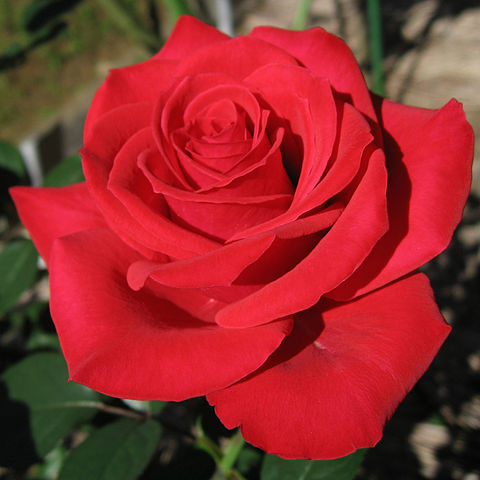# Geometry of Love

Geometry Level 4A boy named Alex plans to give a rose with a beautiful ring to his sweetheart Rosie.

But Rosie likes a specific kind of rose with a ring such that -

If the rose is considered as a 2-D circle , the ring is a point $R$ with coordinates $(-3 , -5)$, the power of the point $R$ (ring) is '101' with respect to the circle(rose). [2- D Geometry]

Alex collects 5 roses with one ring each with coordinates $(-3 , -5)$ and opts to select the appropriate rose, which his sweetheart Rosie would like on Valentine's Day.

The collected roses had the following equations:

Rose R : $x^2 + y^2 - 2x - 4y + 9 = 0$

Rose O : $x^2 + y^2 - 4x - 6y + 9 = 0$

Rose S : $x^2 + y^2 - 6x - 8y + 9 = 0$

Rose I : $x^2 + y^2 - 8x - 10y + 9 = 0$

Rose E :$x^2 + y^2 - 10x - 12y + 9 = 0$

You have to help Alex to select the right rose for his valentine. Which one will you suggest?

Bonus question: Alex and Rosie are the main romantic characters in a film released in 2014. What is the name of this film?

×